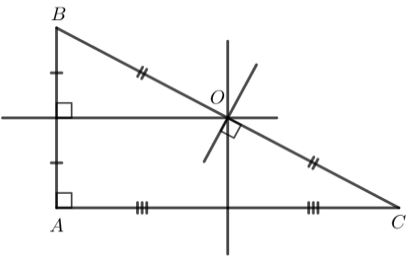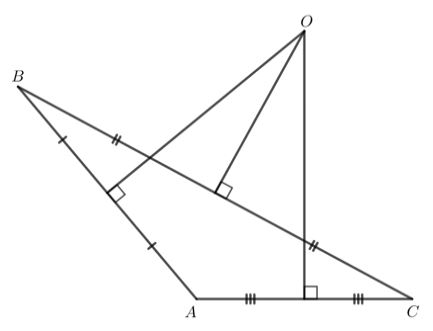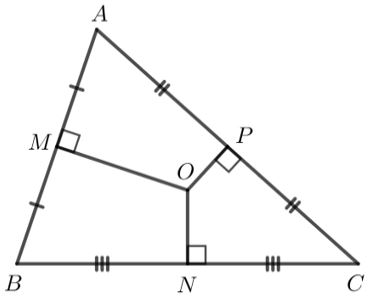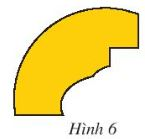## Solution of Exercise 6: Properties of the three perpendicular bisectors of a triangle (C8 Math 7 Horizons) – Math Book

Solution of Exercise 6: Properties of the three perpendicular bisectors of a triangle (C8 Math 7 Horizons)
============

### Solution 1 page 72 Math textbook 7 Creative horizon volume 2 – CTST

Draw three acute, square and obtuse triangles

a) Determine the point O equidistant from the three vertices of each triangle

b) State your comments on the position of point O in each case.

Detailed instructions for solving Lesson 1

Solution method

Draw 3 triangles and determine the point O

Detailed explanation

a) Call the three vertices of the triangle A; B; C.

Point O is equidistant from the three vertices of triangle ABC, so O is the intersection of the three perpendicular bisectors of triangle ABC.

+) Pointed triangle:+) Right triangle:+) Obtuse triangle:b) For an acute triangle, the intersection of the three perpendicular bisectors of the triangle lies within that triangle.

In a right triangle, the intersection of the three perpendicular bisectors is the midpoint of the hypotenuse of the triangle.

For an obtuse triangle, the intersection of the three perpendicular bisectors of the triangle lies outside the triangle.

–>

— *****

### Solution 2 page 72 Math textbook 7 Creative horizon volume 2 – CTST

Let ABC be an acute triangle. Let M, N, P be the midpoints of the sides AB, BC, CA respectively and let O be the point equidistant from the three vertices of the triangle ABC. Prove that MO is perpendicular to AB, NO is perpendicular to BC, and PO is perpendicular to AC.

Detailed instructions for solving Lesson 2

Solution method

We prove that OM, ON, OP are the perpendicular bisectors corresponding to the side of the triangle

Detailed explanationO is a point equidistant from the three vertices of triangle ABC, so O is the intersection of the three perpendicular bisectors of triangle ABC.

Then because M, N, P are the midpoints of sides AB, BC, CA, respectively, so MO

$\perp$

AB, NO

$\perp$

BC, PO

$\perp$

AC.

–>

— *****

### Solution 3 page 72 Math textbook 7 Creative horizon volume 2 – CTST

It was wanted to restore a broken circular disc with only one piece remaining (Figure 6). How to determine the radius of this ancient disk?Detailed instructions for solving Lesson 3

Solution method

Using the property of the intersection of 2 orthogonals in a triangle

Detailed explanation

Let O be the center of the disk

$$\Rightarrow$$ OA = OB = OC = r ( due to the same length = radius )

Consider triangle ABC where O is a point equidistant from A, B, C

$$\Rightarrow$$ O is the intersection of the three perpendicular bisectors of triangle ABC

To determine O, we draw two perpendicular bisectors of AB and BC, they intersect at O.

–>

— *****